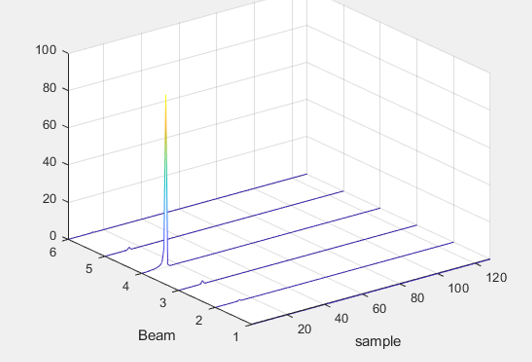FPGA Intellectual Property
PCI Express*, Networking and Connectivity, Memory Interfaces, DSP IP, and Video IP
6223 Discussions

## FFT IP MATLAB C-model seems not bit trueBeginner
537 Views

We included the Intel FFT IP (altera_fft_ii) in our Arria 10 and ran into the following 2 issues.

1. The first issue (bug?) is related to the data representation. The core is configured with “Single Floating Point” data representation.

In our design process, we are using the generated Matlab model to verify the outcome of the HDL simulation.

There seems to be a bug in the Matlab files of the Generated Example Design.

Inside the generated Matlab function fft_ii_o_example_design_model (in fft_ii_0_example_design_model.m) the REPRESENTATION parameter is defined and set to 0.

The value of 0 complies to fixed-point data representation and is therefore incorrect!

After having changed the value manually in 1, the matlab outcome of the FFT is “almost” identical to the HDL simulation outcome.

This brings me to the second issue…

2. Matlab FFT outcome unequal to HDL simulation outcome

We noticed that in our design the Matlab FFT outcome of the FFT was not equal to the HDL simulation outcome of the FFT. I was expecting that the Matlab model would be bit-accurate with the HDL model. Is this assumption correct?

To further investigate the issue, I simulated the HDL testbench of the Generated Example Design and compared the outcome with the outcome of the Matlab testbench. The results are not exactly identical!!!

Outcome Matlab testbench:

.\Matlab_model\fft_ii_0_example_design_real_output_c_model.txt

.\Matlab_model\fft_ii_0_example_design_imag_output_c_model.txt

Outcome HDL testbench:

.\sim\tb\fft_ii_0_example_design_real_output.txt

.\sim\tb\fft_ii_0_example_design_imag_output.txt

Instructions for reproducing the files:

1. ModelSim:
• Go to the Project Directory: ./sim/tb
• In transcript window type:

source mentor.do

2. Matlab:

• Navigate to the directory: .\Matlab_model\
• Run: fft_ii_0_example_design_tb.m

4 RepliesEmployee
320 Views

Hi,

As I understand it, you have some inquires related to the FFT Matlab model simulation output has discrepancies compared to Modelsim simulation. Thanks for the detailed explanation.

To ensure we are on the same page, just would like to check with you on the following:

1. Just would like to check with you which Quartus version and FPGA device that you are using?

2. Would you mind to further elaborate on the difference that you are observing with the output data? Some screenshots and your expectation would be helpful.

3. Just wonder if you are expecting the output values are exactly identical number? If yes, for your information, based on my previous experience, the Matlab output might have difference in term of magnitude vs HDL simulation. This might be due to scaling or rounding difference between the tools and models.

4. Although magnitude might be different, generally the peak frequency would be almost similar. Just wonder if you are observing that the peak frequency are far off between Matlab vs HDL simulation? Or if you are observing the plots have significant difference in shape?

Please let me know if there is any concern. Thank you.

Best regards,

Chee PinBeginner
320 Views

Hi Chee Pin,

1. We are using Quartus Prime version 17.1.1. Internal Build 593 and the target device is Arria 10 (10AS066H2F).
2. In the screenshots below you see for 6 sweeps the FFT outcome (represented as re^2 + im^2) and the associated absolute difference between the HDL simulation results and the Matlab model. Each sweep consists in this case of 128 samples. The max. absolute difference observed in this case is ~5*10^-6 at the peak value of the FFT, which is in this case ~80.3. We were indeed expecting a bit-accurate Matlab model. We have this experience with the fixed-point version of the FFT IP and were also expecting this behavior when using floating-point.

The FFT IP user guide states that the Matlab model is bit-accurate: https://www.intel.com/content/www/us/en/programmable/documentation/hco1419012539637.html4. The peak-frequency seems indeed identical, its magnitude almost...

Kind regards,

Erwin MijnenEmployee
320 Views

Hi,

Sorry for the delay. As I understand it, you are expecting that the bit accurate Matlab model should give the exact output as the Modelsim simulation output. However, from your observation, the floating point output for Matlab model seems has little discrepancy vs the Modelsim output. This seems to be happening for floating point but not fixed point. You are able to replicate this observation with the example design generated by the FFT, the file you share.

Based on my understanding, ideally the bit accurate model should give same output as Modelsim. In this case, I believe it is due to some rounding in the Matlab model. As a workaround, I would recommend you to refer to the Modelsim simulation.

Please feel free to share with me the FFT .qsys or .ip file as well. I would like to file a case to Engineering to look into this and probably for future fix.

Thank you very much.Employee
320 Views

Hi,

Just would like to follow up with you on this. Thank you.